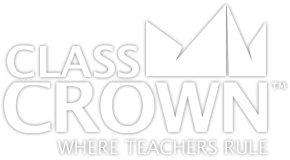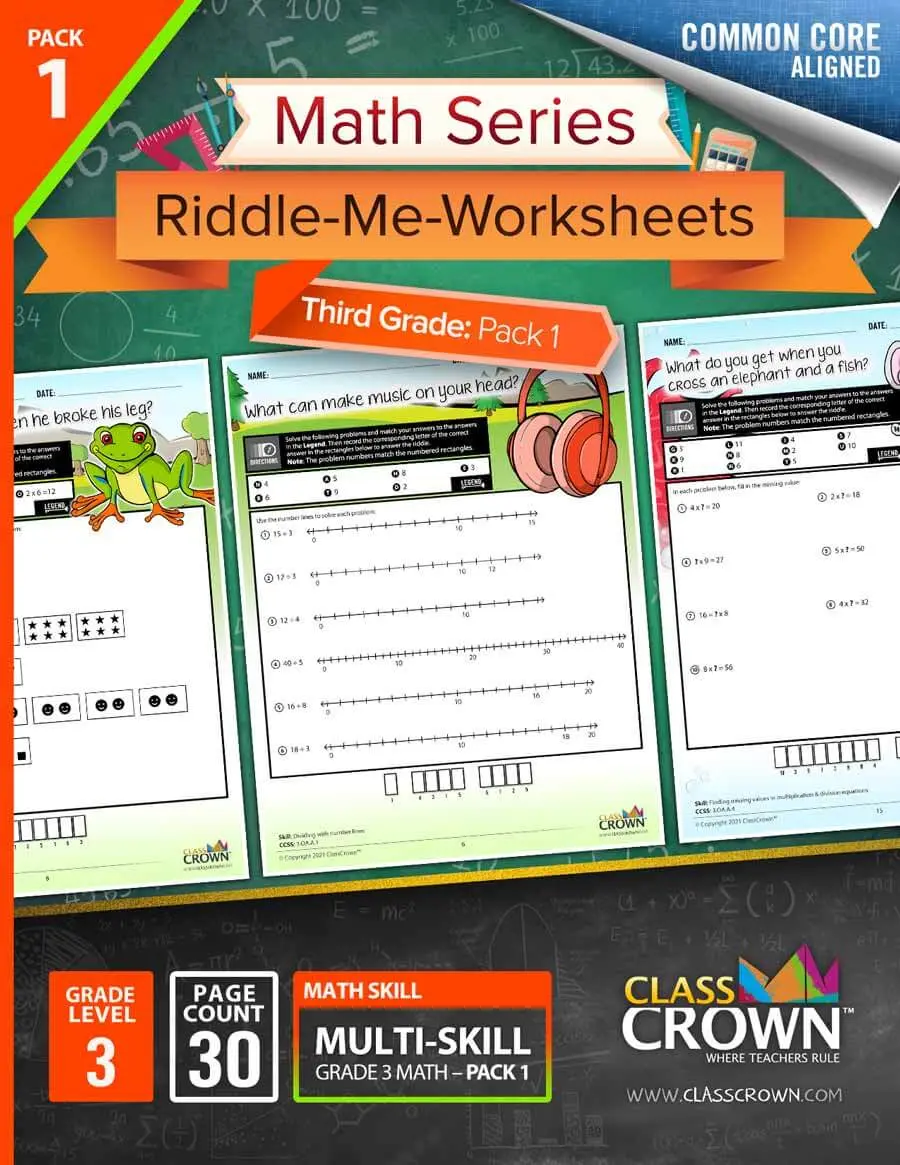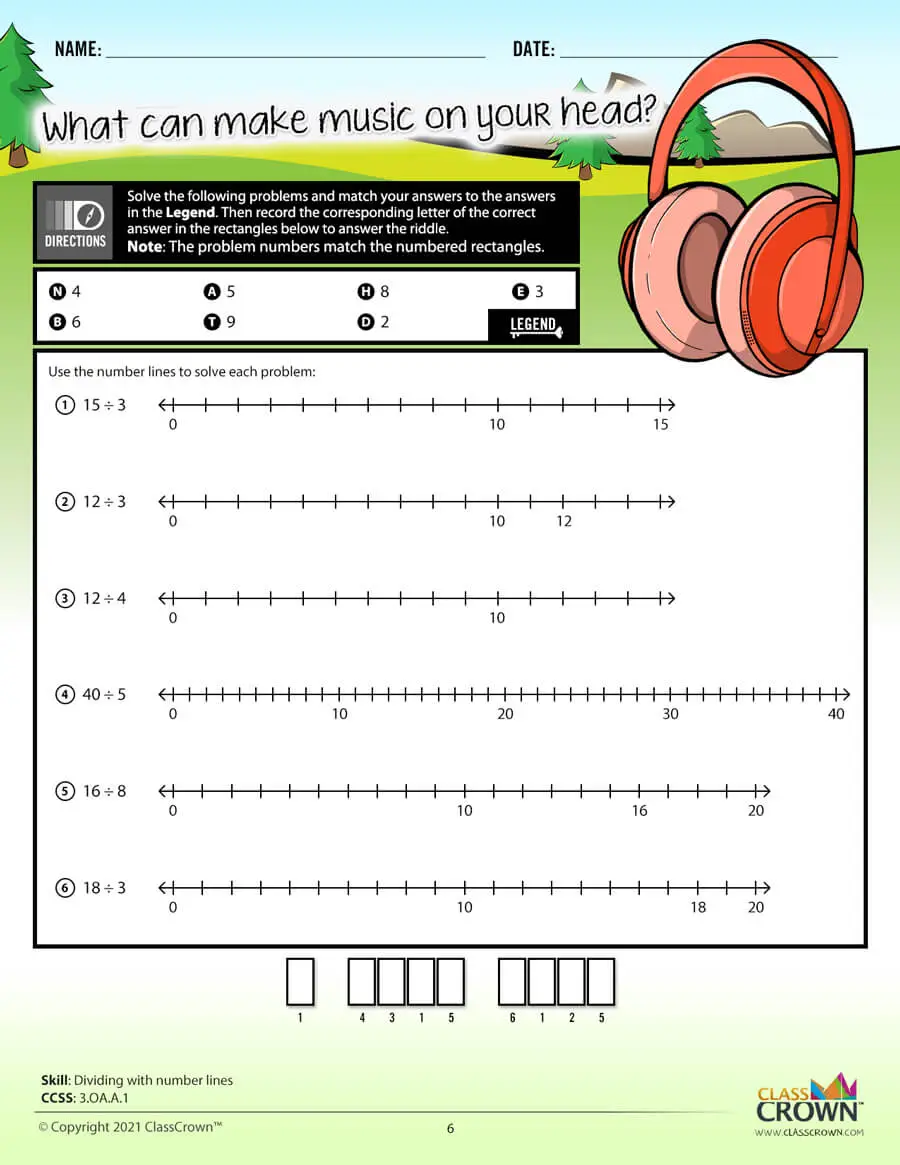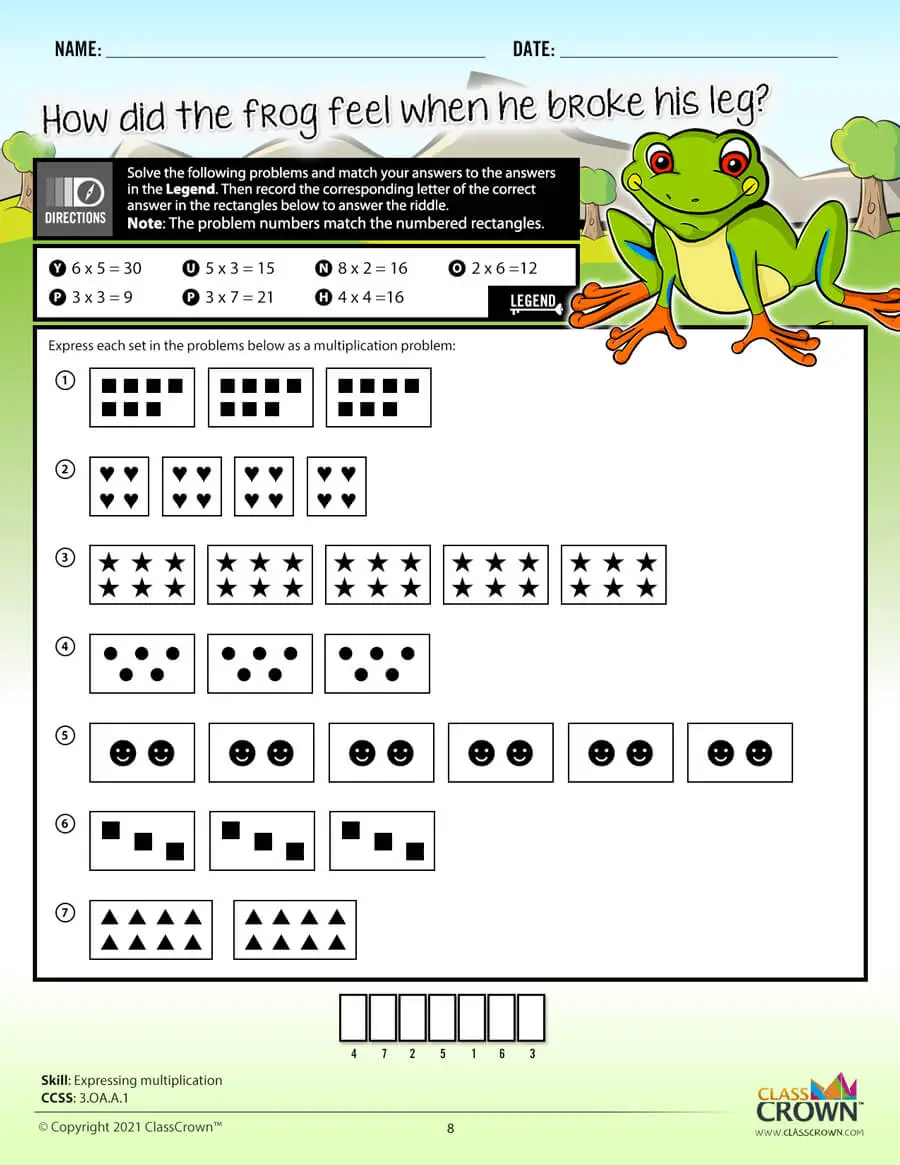Math Worksheets

# 3rd Grade Math Worksheets: Pack 1

## Self-checking math worksheets with riddles to keep kids motivated.

Rating: 0/5
(0 Reviews)
3rd
WORKSHEET COUNT
30
FORMAT(S)
PDF
Yes
\$8.99
This comprehensive bundle includes 3rd grade packs 1–3.
\$20.99
\$26.97
Save 22%
The Details
Description
• Do your students ever complete an entire math worksheet, only to find that they have done most of the problems incorrectly and clearly have a misconception?
• ClassCrown’s Riddle-Me-Worksheets help to prevent this problem by introducing a puzzle aspect to math, giving students immediate feedback as to whether or not they are solving problems correctly. If the answer to the riddle isn't correct, the student knows which problems he's made an error on.
Specifics
• Subject:  Division, Multipliation, Number Lines +more!
• Product Count:  30
• Full Page Count:  51
• File Format(s):  PDF

What's Included?
• Dividing with number lines
• Multiplying with number lines
• Expressing multiplication
• Understanding multiplying by 10s
• Interpreting whole number division
• Interpreting arrays as multiplication
• Multiplying within 100, word problems
• Finding a missing fact in multiplication/division
• Finding missing values in multiplication & division equations
• Completing the associative property
• Finding associative property of multiplication
• Finding communicative property of multiplication
• Identifying distributive property of multiplication
• Using distributive property
• Division relative to multiplication
• Completing multiplication/division charts
• Multiplying within 100
• Dividing within 100
• Multiplying and dividing within 100
• Finding missing value in multiplication and division within 100
• Dividing in word problems (one digit quotient, no remainder)
• Dividing in word problems (one digit quotient with remainder)
• Solving 2-step word problems (add and then divide)
• Solving 2-step word problems (add and multiply)
• Determining which expression represents the solution to a word problem
• Solving 2-step word problems (multiply then add)
• Solving 2-step word problems (subtract & divide)
• Solving 2-step word problems (subtract and multiply)
• Determining rule in a function table
CCSS: 3.OA.A.1, 3.OA.A.2, 3.OA.A.3, 3.OA.A.4, 3.OA.A.4.1, 3.OA.A.5, 3.OA.A.6, 3.OA.A.7, 3.OA.A.8, 3.OA.A.9
Reviews
Overall Rating: 0/5
(Showing 0 of 0)
See More Reviews @ TpTSelf Checking

Our math worksheets introduce a puzzle aspect to math, giving students immediate feedback as to whether or not they are solving problems correctly. If the answer to the riddle isn't spelled correctly, the student knows which problems he's made an error on.Fun Puzzle AspectImmediate Feedback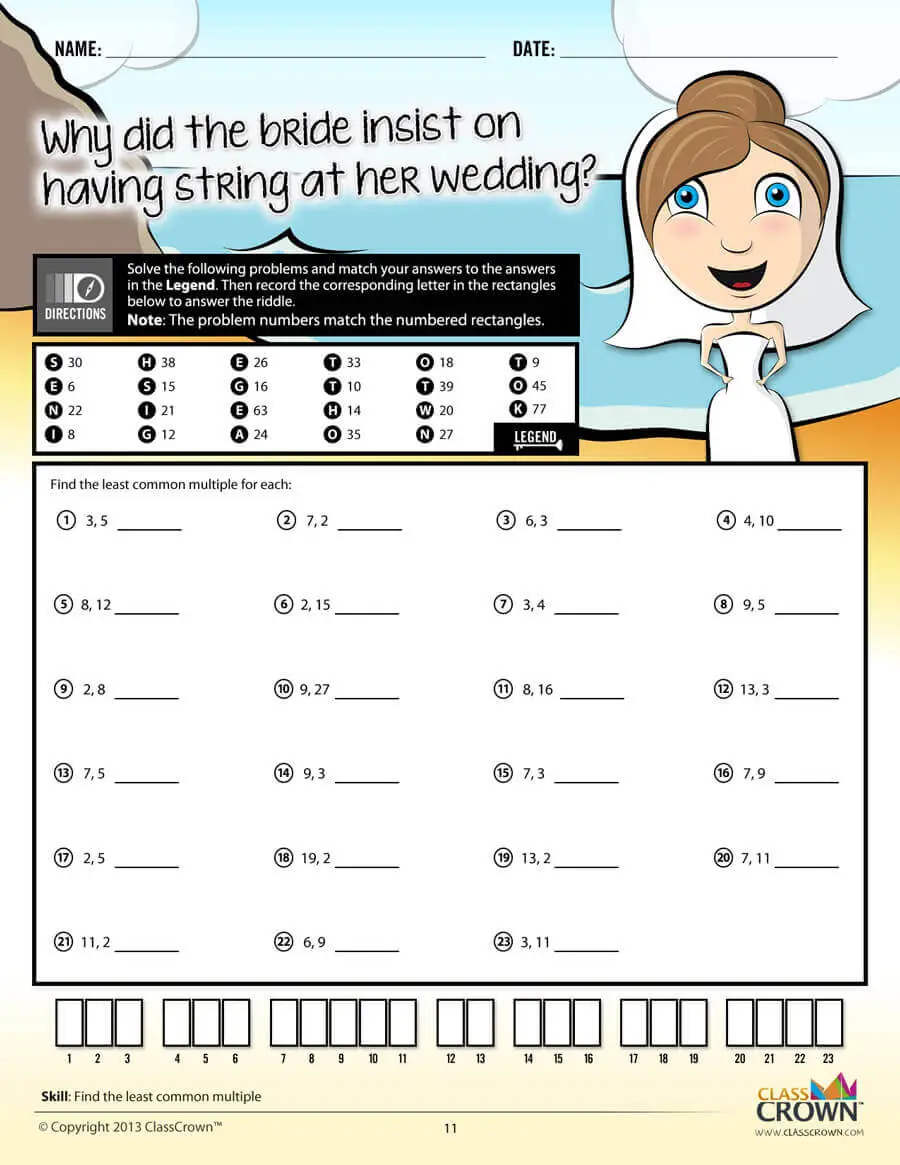Problem Solving Motivation

Each math worksheet contains a riddle that the student solves by completeing all the problems on the worksheet. This keeps kids motivated to complete each problem so that they can find the answer to the riddle.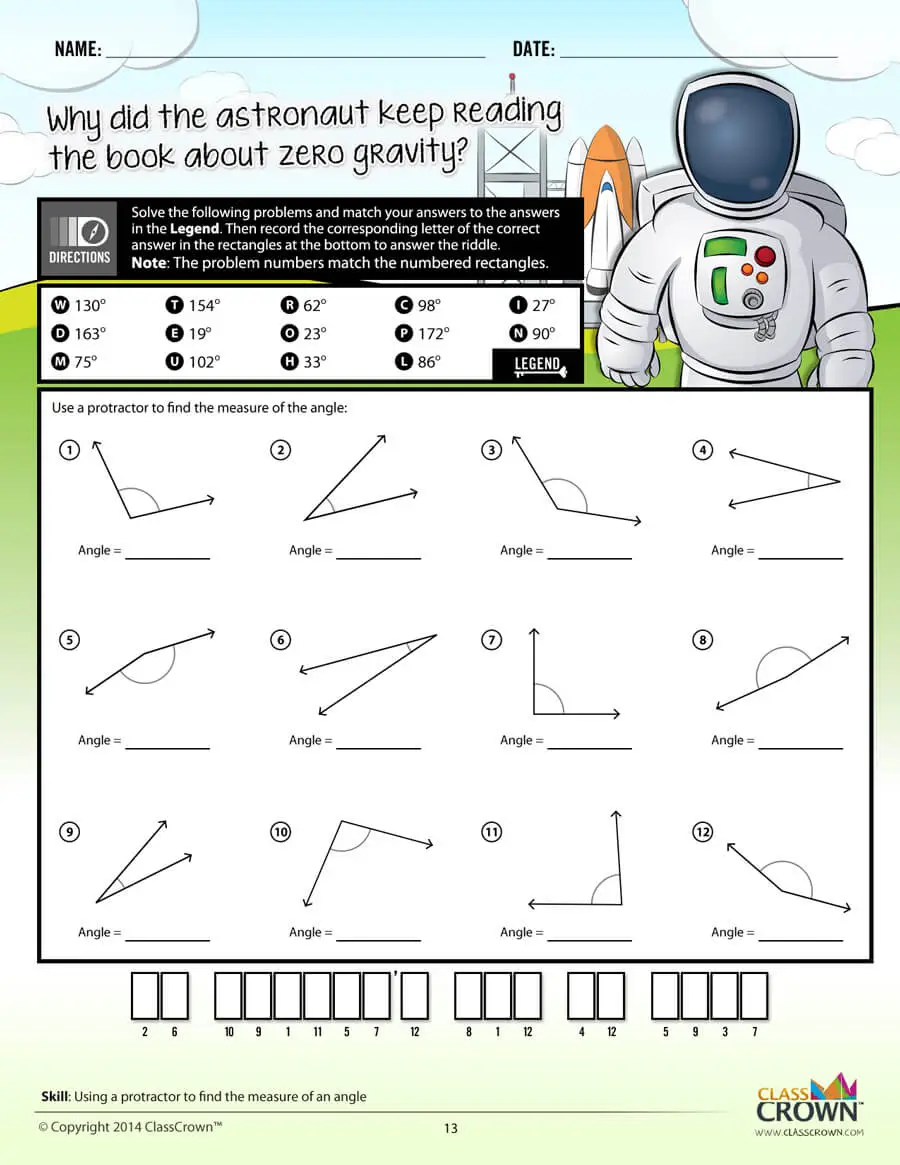Common Core Aligned

All our math worksheet packs are designed with Common Core in mind. That way you don’t have to worry about whether your math ciriculum is aligned or not when you incorpoate ClassCrown Riddle-Me-Worksheets in your lesson plans.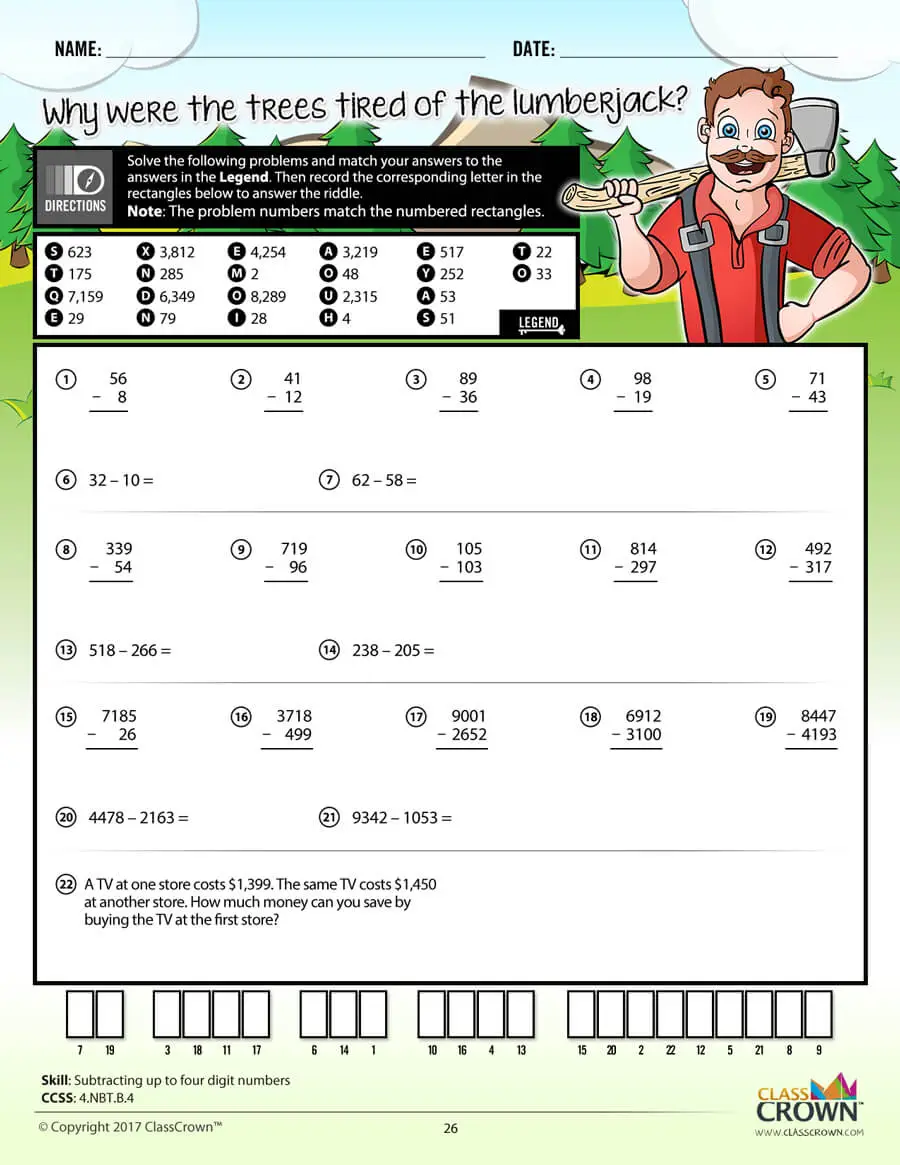High Quality Design

Each page of our math worksheets has been produced in high resolution at 144 dpi and designed in full, vibrant color for maximum quality. They look stunning whether you are printing in color or black and white.High Resolution
(144 dpi)Stunning Color & Clarity